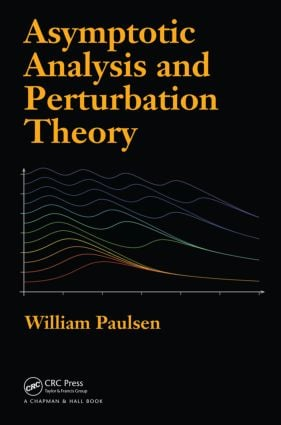Asymptotic Analysis and Perturbation Theory

1st Edition

Chapman and Hall/CRC

550 pages | 104 B/W Illus.

Hardback: 9781466515116
pub: 2013-07-18
\$150.00
x
eBook (VitalSource) : 9780429189531
pub: 2013-07-18
from \$28.98

FREE Standard Shipping!

Description

Beneficial to both beginning students and researchers, Asymptotic Analysis and Perturbation Theory immediately introduces asymptotic notation and then applies this tool to familiar problems, including limits, inverse functions, and integrals. Suitable for those who have completed the standard calculus sequence, the book assumes no prior knowledge of differential equations. It explains the exact solution of only the simplest differential equations, such as first-order linear and separable equations.

With varying levels of problems in each section, this self-contained text makes the difficult subject of asymptotics easy to comprehend. Along the way, it explores the properties of some important functions in applied mathematics. Although the book emphasizes problem solving, some proofs are scattered throughout to give readers a justification for the methods used.

Introduction to Asymptotics

Basic Definitions

Limits via Asymptotics

Asymptotic Series

Inverse Functions

Dominant Balance

Asymptotics of Integrals

Integrating Taylor Series

Repeated Integration by Parts

Laplace's Method

Review of Complex Numbers

Method of Stationary Phase

Method of Steepest Descents

Speeding Up Convergence

Shanks Transformation

Richardson Extrapolation

Euler Summation

Borel Summation

Continued Fractions

Differential Equations

Classification of Differential Equations

First Order Equations

Taylor Series Solutions

Frobenius Method

Asymptotic Series Solutions for Differential Equations

Behavior for Irregular Singular Points

Full Asymptotic Expansion

Local Analysis of Inhomogeneous Equations

Local Analysis for Nonlinear Equations

Difference Equations

Classification of Difference Equations

First Order Linear Equations

Analysis of Linear Difference Equations

The Euler-Maclaurin Formula

Taylor-Like and Frobenius-Like Series Expansions

Perturbation Theory

Introduction to Perturbation Theory

Regular Perturbation for Differential Equations

Singular Perturbation for Differential Equations

Asymptotic Matching

WKBJ Theory

The Exponential Approximation

Region of Validity

Turning Points

Multiple-Scale Analysis

Strained Coordinates Method (Poincaré-Lindstedt)

The Multiple-Scale Procedure

Two-Variable Expansion Method

Appendix: Guide to the Special Functions

Bibliography

Index